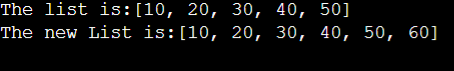## Introduction:

LinkedList is a linear data structure that is used to store the data. There is a very famous and useful method for LinkedList add() method. This method is used to add the data to our LinkedList.

There are two ways to apply this method.

Let us discuss each of them:-

Using the above method we can add the data at the end of the LinkedList.

We need to pass the data to this method which we need to add to our LinkedList. This method appends the data at the end of the LinkedList. The above method returns true if the element is appended to the LinkedList.

Refer to the below code for more understanding.

Output:Time Complexity: The time complexity for the above functions is O(1).

Space Complexity: The space complexity for the above method is O(N) to maintain all the ‘N’ elements of the LinkedList.

Using the above method we can add the data at any particular index of the LinkedList.

Syntax: boolean add(int index, Object X)

The above method returns true if at least one element is appended to the LinkedList at the given index.

Refer to the below code for more understanding.

Output:Time Complexity: The time complexity for the above functions is O(N) because we need to traverse to the given index.

Space Complexity: The space complexity for the above method is O(N) to maintain all the N elements of the LinkedList.

## FAQs:

1. What are the two types of LinkedList add() methods we can use?
2. What is the time complexity for both functions?
• The time complexity for the first function is O(1), whereas the Time complexity for the second method is O(N) because we need to traverse to the given index.

## Key Takeaways:

In this blog, we have covered the following things:

2. Then we discussed the two types of LinkedList add method in Java.

Until then, All the best for your future endeavors, and Keep Coding.